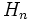# Expected number of cycles of permutation equals harmonic number of degree

(diff) ← Older revision | Latest revision (diff) | Newer revision → (diff)

## Statement

Suppose$n$ is a natural number. Consider the uniform distribution on the symmetric group of degree$n$. Then, the expected number of cycles in the cycle decomposition of a permutation chosen according to the uniform distribution is equal to the harmonic number$H_n$ of$n$, where:$H_n := 1 + \frac{1}{2} + \frac{1}{3} + \dots + \frac{1}{n}$

Note that$\lim_{n \to \infty} H_n - \log n = \gamma$, where$\gamma$ is the Euler-Mascheroni constant, and its value is approximately$0.577$ (or very close to$1/\sqrt{3}$). Also,$\lim_{n \to \infty} H_n/\log n = 1$. Thus, for$n$ large enough,$H_n$ can be approximated additively as$\gamma + \log n$ and multiplicatively as$\log n$.

## Particular cases$n$$H_n$ (equals expected number of cycles)$\log n$
1 1 0
2 1.5 = 3/2 0.6931...
3 1.833.. = 11/6 1.0986...
4 2.0833.. = 25/12 1.3862...
5 2.2833.. = 137/60 1.6094...

## Relation with Stirling numbers of the first kind

Denote by$s(n,k)$ the unsigned Stirling number of the first kind, defined as the number of permutations of$\{ 1,2,3,\dots,n\}$ with$k$ cycles. The expected number of cycles of a permutation can then be computed as:$\sum_{k=1}^n \frac{ks(n,k)}{n!}$$\sum_{k=1}^n \frac{ks(n,k)}{n!} = H_n$

## Proof

For the prooof, we will work with the symmetric group on the set$\{ 1,2,3,\dots,n \}$ for concreteness. However, the result applies to the expected number of cycles for the symmetric group on any set of size$n$.

### Review of the Foata transformation

The standard proof of the statement uses the Foata transformation. The Foata transformation is a bijection from$S_n$ to itself that relies on the "pun" between cycle decompositions and one-line notation. The steps are as follows:

1. Write the permutation in canonical cycle decomposition notation: By the cycle decomposition theorem, the permutation has a cycle decomposition. Write the cycle decomposition in canonical notation, including fixed points. Make sure to include fixed points as cycles of size one. Explicitly, this means:
• Cyclically rearrange each cycle so that it begins with its largest element.
• Arrange the cycles in increasing order of their largest elements.
2. Reinterpret in one-line notation: Now, drop the commas and parentheses and you get a string of length$n$ that is the one-line notation for a permutation of$\{ 1,2,3,\dots,n\}$(more explicitly, it is the second line of the two-line notation of the permutation of$\{1,2,3,\dots,n\}$, where the first line is$1,2,3,\dots,n$). This is the new permutation that is the image of the Foata transformation.

The inverse of this bijection is as follows:

1. Write the permutation in one-line notation (i.e., write it as a string whose$i^{th}$ element is the image of$i$ under the permutation).
2. Traverse through the string from left to right, and identify all the left-to-right maxima, i.e., all the elements that are larger than the elements before them. The strings starting with a given left-to-right maxima and ending just before the next form the strings for the cycles in the canonical notation for the cycle decomposition of the original permutation.

### Counting the number of cycles using the Foata transformation

From the above, we obtain that:

Number of cycles of a permutation = Number of left-to-right maxima in the image of that permutation under the Foata transformation

Applying this to the uniform probability distribution over$S_n$, we obtain:

Expected number of cycles of a permutation = Expected number of left-to-right maxima of a permutation in one-line notation

By the additivity of expectation, the right side can be simplified as:$\sum_{i=1}^n$ (Expected number of left-to-right maxima at the$i^{th}$ position)

The summands are now simply probabilities. So the summation becomes:$\sum_{i=1}^n$ (Probability that the entry in the$i^{th}$ position of a permutation is a left-to-right maximum)

This probability is$1/i$: intuitively, what's happening is that, once we decide what elements to choose for the first$i$, then exactly$1/i$ of the permutations with those elements will have the largest among them in the last ($i^{th}$ position). Thus, we get that the expected number of cycles in a permutation is:$\sum_{i=1}^n \frac{1}{i}$

### Comment on independence of probabilities

The expected number of left-to-right maxima is the sum of the probabilities, for each position, that the entry in that position is a left-to-right maximum. In order to make this observation, we do not need to demonstrate that the probabilities are independent of one another. However, it turns out that the probabilities are indeed independendent of one another.